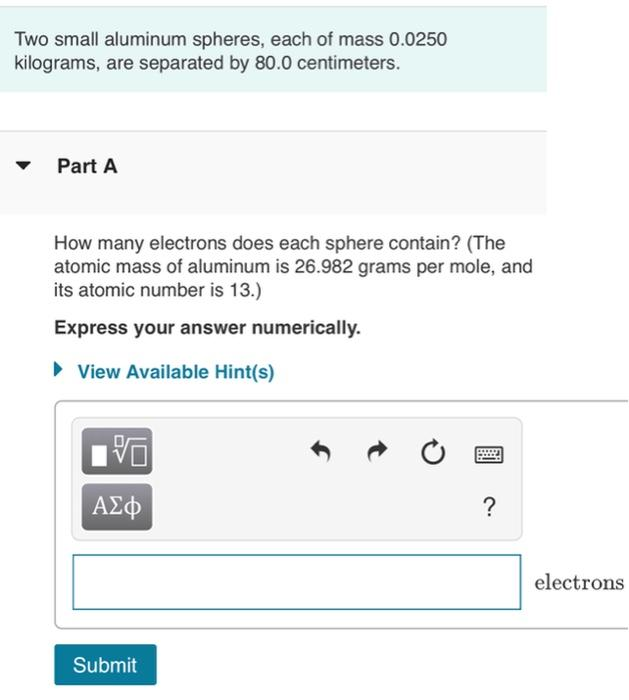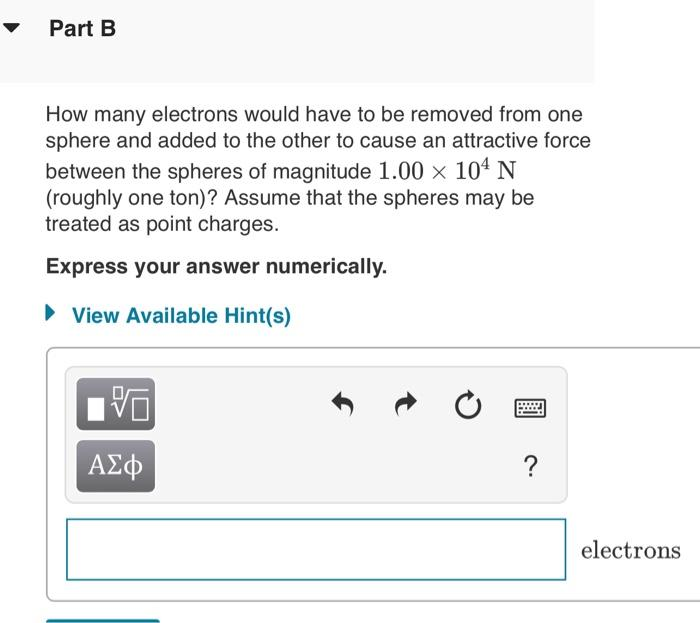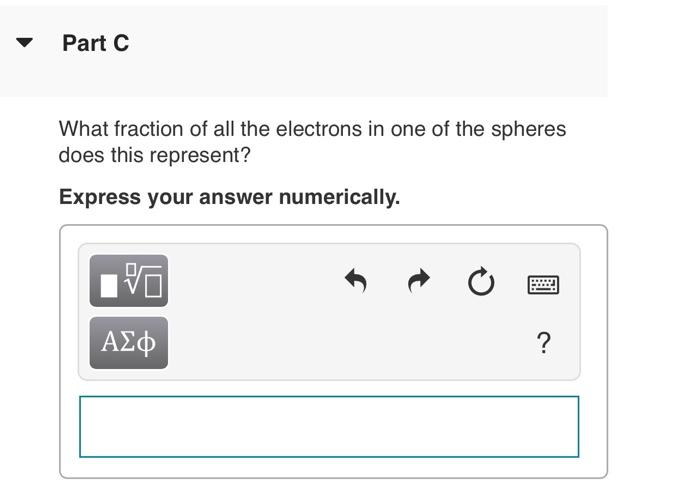# Question Solved1 AnswerAnswer all parts for a rate! Two small aluminum spheres, each of mass 0.0250 kilograms, are separated by 80.0 centimeters. Part A How many electrons does each sphere contain? (The atomic mass of aluminum is 26.982 grams per mole, and its atomic number is 13.) Express your answer numerically. How many electrons would have to be removed from one sphere and added to the other to cause an attractive force between the spheres of magnitude 1.00×104 N (roughly one ton)? Assume that the spheres may be treated as point charges. Express your answer numerically. electrons What fraction of all the electrons in one of the spheres does this represent? Express your answer numerically.Answer all parts for a rate!Transcribed Image Text: Two small aluminum spheres, each of mass 0.0250 kilograms, are separated by 80.0 centimeters. Part A How many electrons does each sphere contain? (The atomic mass of aluminum is 26.982 grams per mole, and its atomic number is 13.) Express your answer numerically. How many electrons would have to be removed from one sphere and added to the other to cause an attractive force between the spheres of magnitude 1.00×104 N (roughly one ton)? Assume that the spheres may be treated as point charges. Express your answer numerically. electrons What fraction of all the electrons in one of the spheres does this represent? Express your answer numerically.
More
Transcribed Image Text: Two small aluminum spheres, each of mass 0.0250 kilograms, are separated by 80.0 centimeters. Part A How many electrons does each sphere contain? (The atomic mass of aluminum is 26.982 grams per mole, and its atomic number is 13.) Express your answer numerically. How many electrons would have to be removed from one sphere and added to the other to cause an attractive force between the spheres of magnitude 1.00×104 N (roughly one ton)? Assume that the spheres may be treated as point charges. Express your answer numerically. electrons What fraction of all the electrons in one of the spheres does this represent? Express your answer numerically.